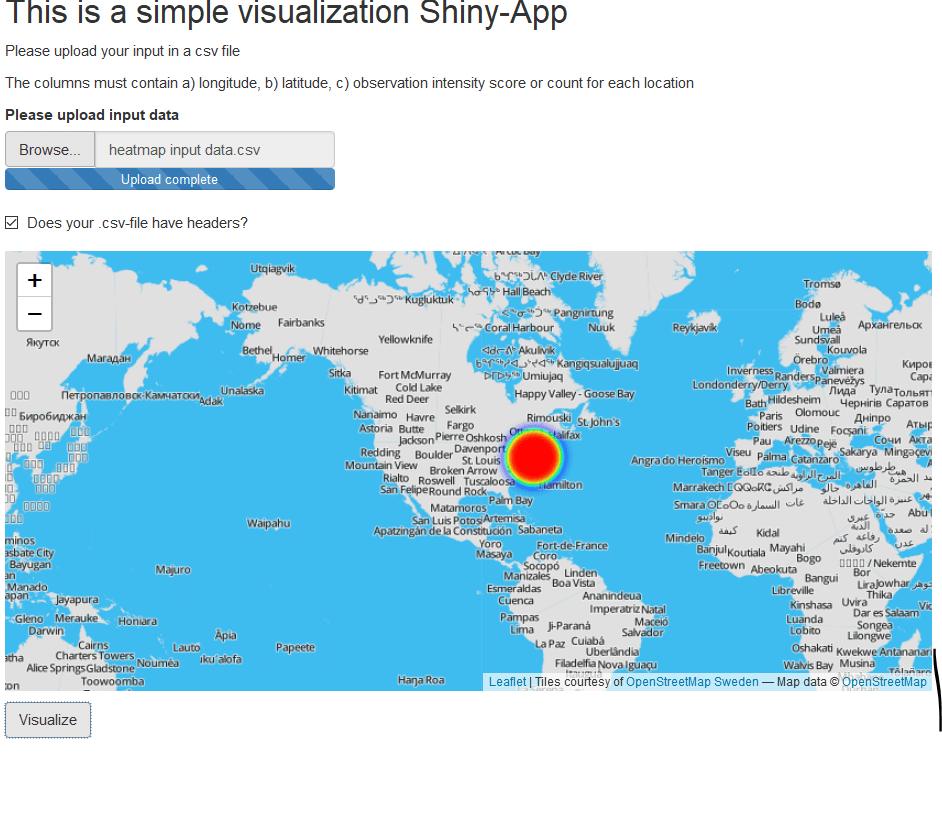# Coding a Leaflet Shiny App for drawing heatmapsIn previous posts I have demonstrated how to create heatmaps in R, using e.g. deckgl, ggmap and Leaflet. I have also given an overview of several packages applicable for the purpose of visualizing spatial data in R.

This post provides a brief coding example of how to program a small Shiny App in R, implementing the Leaflet package for visualizing heatmaps on Leaflet map tiles.

The code for this is as follows:

``````# loading required packages
library(shiny)
library(leaflet)
library(leaflet.extras)
library(jsonlite)

# GRAPHICAL USER INTERFACE
ui <- fluidPage(
titlePanel("This is a simple visualization Shiny-App"),
p("The columns must contain a) longitude, b) latitude, c) observation intensity score or count for each location"),
leafletOutput(outputId = "map_result"),
p(""),
actionButton(inputId="input_button",label="Visualize")
)

# SERVER
server <- function(input,output){
output\$map_result <- renderLeaflet({
# call to action button
input\$input_button

# read and process input data
isolate({
data_file <- req(input\$input_file)
if(is.null(data)){
return(NULL)
}else{
}

# visualize output data
leaflet() %>%
setView(lng= mean(data[,1]), lat=mean(data[,2]), zoom=6) %>%
addHeatmap(lng =data[,1] , lat=data[,2] , intensity=data[,3])
})
})
}

# APP shinyApp(ui=ui,
server=server)
``````

The app requests an csv file as data source. This csv file must contain longitude and latitude data in columns, for each location that should be considered by the heatmap drawing. In addition, the csv file must contain the observation. Below you see an exemplaric screenshot: